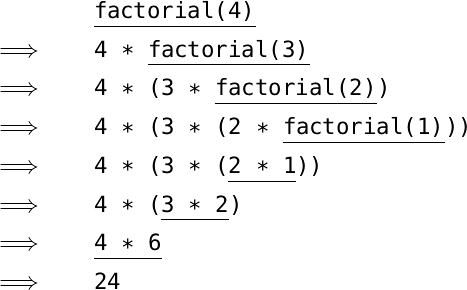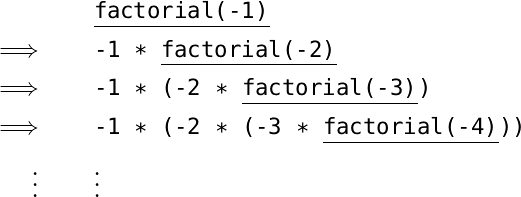Back to Contents

# 2. Names and Functions

So far we have built only tiny toy programs. To build bigger ones, we need to be able to name things so as to refer to them later. We also need to write expressions whose result depends upon one or more other things.

### Names

So far, if we wished to use a sub-expression twice or more in a single expression, we had to type it multiple times:

``````Python
>>> 200 * 200 * 200
8000000``````

Instead, we can define our own name to stand for the result of evaluating an expression, and then use the name as we please:

``````Python
>>> x = 200
>>> x * x * x
8000000``````

We can update the value associated with the name and try the calculation again:

``````Python
>>> x = 200
>>> x * x * x
8000000
>>> x = 5 + 5
>>> x * x * x
1000``````

Because of this ability to vary the value associated with the name, things like `x` are called variables. We can use any name we like for a variable, so long as it does not clash with any of Python’s built in keywords:

`and as assert async await break class continue def del elif else except finally for from global if import in is lambda nonlocal not or pass raise return try while with yield`

In Python, we use lower case letters or words for variable names. For example `x`, `weight`, or `total`. If we wish to use multiple words, we separate them with underscores. For example `first_string` or `total_of_subtotals`.

### Functions

We can make a function, whose value depends upon some input. We call this input an argument – we will be using the word “input” later in the book to mean something different:

``````Python
>>> def cube(x): return x * x * x
...
>>> cube(10)
1000
8000``````

Note that we had to press the Enter key twice when defining the function: we shall discover why momentarily. What are the parts to this definition of the function `cube`? We write `def`, followed by the function name, its argument in parentheses, and a colon. Then, we calculate `x * x * x` and use `return` to return the value to us.

We need the word `return` because not all functions return something. For example, this function prints a string given to it twice to the screen, but does not return a value:

``````Python
>>> def print_twice(x):
...     print(x)
...     print(x)
...
>>> print_twice('Ha')
Ha
Ha
>>> print_twice(1)
1
1``````

Notice this function spans multiple lines. It can operate on both strings and numbers. Now you can see why we needed to press Enter twice when defining the `cube` and `print_twice` functions – so that Python knows when we have finished entering a multi-line function.

### Indentation

Each of the `print(x)` lines in `print_twice` is indented (moved to the right by insertion of four spaces). This helps us to show the structure of the program more clearly, and in fact is a requirement – Python will complain if we do not do it:

``````Python
>>> def print_twice(x):
... print(x)
File "<stdin>", line 2
print(x)
^
IndentationError: expected an indented block``````

You will come across this error frequently as you learn to indent your Python programs correctly.

### Functions with choices

We can use the keywords `if` and `else` to build a function which makes a choice based on some test. For example, here is a function which determines if an integer is negative:

``````Python
>>> def neg(x):
...     if x < 0:
...         return True
...     else:
...         return False``````

We can test it like this:

``````Python
>>> neg(1)
False
>>> neg(-1)
True``````

Notice the indentation of each part of this function, after every line which ends with a colon – again, it is required. We can write it using fewer lines, if it will fit:

``````Python
>>> def neg(x):
...     if x < 0: return True
...     else: return False``````

Of course, our function is equivalent to just writing

``````Python
>>> def neg(x):
...     return x < 0``````

because `x < 0` will evaluate to the appropriate boolean value on its own – `True` if x < 0 and `False` otherwise. Here is another function, this time to determine if a given string is a vowel or not:

``````Python
>>> def is_vowel(s):
...     return s == 'a' or s == 'e' or s == 'i' or s == 'o' or s == 'u'
>>> is_vowel('x')
False
>>> is_vowel('u')
True``````

If we need to test for more than one condition we can use the `elif` keyword (short for “else if”):

``````Python
>>> def sign(x):
>>>     if x < 0: return -1
>>>     elif x == 0: return 0
>>>     else: return 1``````

This function returns the sign of a number, irrespective of its magnitude. Of course, this could be written without `elif`. Can you see how?

### Multiple arguments

There can be more than one argument to a function. For example, here is a function which checks if two numbers add up to ten:

``````Python
...     return a + b == 10
True
False``````

The result is a boolean. We use the function in the same way as before, but writing two numbers this time, one for each argument the function expects. Finally, let us use the `+` operator in a different way, to concatenate strings:

``````Python
>>> def welcome(first, last):
...     print('Welcome, ' + first + ' ' + last + '! Enjoy your stay.')
>>> welcome('Richard', 'Smith')
Welcome, Richard Smith! Enjoy your stay.``````

### Going round again

A recursive function is one which uses itself in its own definition. Consider calculating the factorial of a given number – for example the factorial of 4 (written 4! in mathematics) is 4 × 3 × 2 × 1. Here is a recursive function to calculate the factorial of a positive number.

``````Python
>>> def factorial(a):
...     if a == 1:
...         return 1
...     else:
...         return a * factorial(a - 1)``````

For example:

``````Python
>>> factorial(4)
24
>>> factorial(100)
933262154439441526816992388562667004
907159682643816214685929638952175999
932299156089414639761565182862536979
208272237582511852109168640000000000
00000000000000``````

How does the evaluation of `factorial(4)` proceed?For the first three steps, the `else` part of the conditional expression is chosen, because the argument `a` is greater than one. When the argument is equal to one, we do not use `factorial` again, but just evaluate to `1`. The expression built up of all the multiplications is then evaluated until a value is reached: this is the result of the whole evaluation. It is sometimes possible for a recursive function never to finish – what if we try to evaluate `factorial(-1)`?The expression keeps expanding, and the recursion keeps going. Helpfully, Python tells us what is going on:

``````Python
>>> factorial(-1)
Traceback (most recent call last):
File "<stdin>", line 1, in <module>
File "<stdin>", line 5, in factorial
File "<stdin>", line 5, in factorial
File "<stdin>", line 5, in factorial
[Previous line repeated 995 more times]
File "<stdin>", line 2, in factorial
RecursionError: maximum recursion depth exceeded in comparison``````

We do not use recursive functions often in Python, preferring the methods of repeated action described in the next chapter. But it can be interesting to think about how they work, and some of the questions at the end of the chapter invite you to do just that.

Almost every program we write will involve functions such as those shown in this chapter, and many larger ones too – using functions to split up a program into small, easily understandable chunks is the basis of good programming.

## Common problems

Now that we are writing slightly larger programs which might span multiple lines, new types of mistake are available to us. A common mistake is to forget the colon at the end of a line. For example, here we forget it after an `if`:

``````Python
>>> def neg(x):
...     if x < 0
File "<stdin>", line 2
if x < 0
^
SyntaxError: invalid syntax``````

Syntax is a word for the arrangement of symbols and words to make a valid program. If we forget the proper indentation, Python complains too:

``````Python
>>> def neg(x):
...     if x < 0:
...     return True
File "<stdin>", line 3
return True
^
IndentationError: expected an indented block``````

We must also remember to avoid using one of Python’s keywords as a variable or function name, even in an otherwise valid program:

``````Python
>>> def class(x): return '30 pupils'
File "<stdin>", line 1
def class(x): return '30 pupils'
^
SyntaxError: invalid syntax``````

Another common mistake is to omit the `return` in a function:

``````Python
>>> def double(x): x * 2
...
>>> double(5)
>>>``````

In this case, Python accepts the function, and we only discover our mistake when we try to use it.

## Summary

We have learned how to give names to our values so as to use and reuse them in different contexts, and to update the values associated with such names. We have written functions whose result depends upon one or more arguments, including multi-line functions. We have seen how to choose a course of action based upon testing the value associated with a name.

Finally, we have experimented with recursive functions to perform repeated processing of one or more arguments. We have explained, though, that recursion is not ordinarily used in Python. In the next chapter, we will introduce the standard Python mechanisms for repeated actions or calculations.

## Questions

Questions 5–8 are optional – we do not often use recursive functions in Python.

1. Write a function which multiplies a given number by ten.

2. Write a function which returns `True` if both of its arguments are non-zero, and `False` otherwise.

3. Write a function `volume` which, given the width, height, and depth of a box, calculates its volume. Write another function `volume_ten_deep` which fixes the depth at 10. It should be implemented by using your `volume` function.

4. Write a function `is_consonant` which, given a lower-case letter in the range `’a’``’z’`, determines if it is a consonant.

5. Can you suggest a way of preventing the non-termination of the `factorial` function in the case of a zero or negative argument?

6. Write a recursive function `sum_nums` which, given a number n, calculates the sum 1 + 2 + 3 + … + n.

7. Write a recursive function `power(x, n)` which raises `x` to the power `n`.

8. Write a recursive function to list the factors of a number. For example, `factors(12)` should print:

``````1
2
3
4
6
12``````